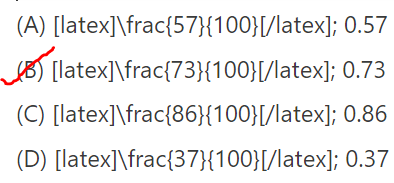Refer to our Texas Go Math Grade 4 Answer Key Pdf to score good marks in the exams. Test yourself by practicing the problems from Texas Go Math Grade 4 Module 2 Assessment Answer Key.

Vocabulary

• decimal
• decimal point
• hundred
• hundredth

Choose the best term from the box to complete the sentence.

Question 1.
A symbol used to separate the ones and the tenths place is called a ______________ .
We know that,
A symbol used to separate the ones and the tenth place is called a “Decimal point”
Hence, from the above,
We can conclude that
The best term that is suitable for the given statement is: Decimal point

Question 2.
The number 0.4 is written as a _____________ .
We know that,
The number 0.4 is written as a “Decimal”
Hence, from the above,
We can conclude that
The best term that is suitable for the given statement is: Decimal

Question 3.
A ______________ is one of one hundred equal parts of a whole.
We know that,
A “Hundredth” is one of one hundred equal parts of a whole.
Hence, from the above,
We can conclude that
The best term that is suitable for the given statement is: Hundredth

Concepts and Skills

Complete the sentence.

Question 4.
0.04 is $$\frac{1}{10}$$ of ____
We know that,
$$\frac{1}{10}$$ × x = y
So,
$$\frac{1}{10}$$ × x = 0.04
x = 0.04 × 10
x = 0.4
Hence, from the above,
We can conclude that
0.04 is $$\frac{1}{10}$$ of 0.4

Question 5.
0.6 is 10 times as much as _____________
We know that,
10 × x = y
So,
10 × x = 0.6
x = 0.6 × $$\frac{1}{10}$$
x = 0.06
Hence, from the above,
We can conclude that
0.6 is 10 times as much as 0.06

Write the number in two other ways.

Question 6.
2.0 + 0.5 + 0.04
The given Expanded form is:
2.0 + 0.5 + 0.04
Now,
The representation of the Standard form for the given Expanded form is: 2.54
The representation of the Word from for the given Expanded from is:
Two and Fifty-four hundredths
Hence, from the above,
We can conclude that
The representation of the Standard form for the given Expanded form is: 2.54
The representation of the Word from for the given Expanded from is:
Two and Fifty-four hundredths

Question 7.
twelve hundredths
The given Word form is:
Twelve hundredths
Now,
The representation of the Standard form for the given Word form is: 0.12
The representation of the Expanded from for the given Word form is:
0 + 0.1 + 0.02
Hence, from the above,
We can conclude that
The representation of the Standard form for the given Word form is: 0.12
The representation of the Expanded from for the given Word form is:
0 + 0.1 + 0.02

Write the fraction or mixed number and the decimal shown by the model.

Question 8.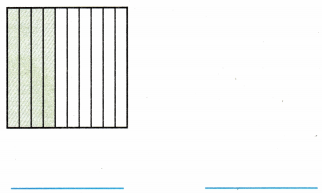The given model is:Now,
From the above model,
We can observe that
The number of shaded parts is: 4
The number of total parts is: 10
So,
The representation of the given model in the form of a fraction is: $$\frac{4}{10}$$
The representation of the given model in the form of a decimal number is: 0.4
Hence, from the above,
We can conclude that
The representation of the given model in the form of a fraction is: $$\frac{4}{10}$$
The representation of the given model in the form of a decimal number is: 0.4

Question 9.The given model is: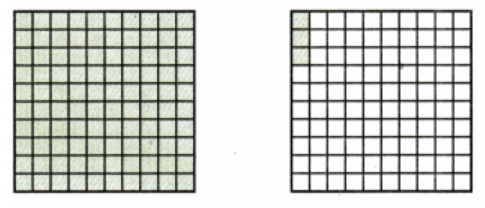Now,
From the above model,
We can observe that
The number of shaded parts is: 103
The total number of parts are: 100
So,
The representation of the given model in the form of a fraction is: $$\frac{103}{100}$$
The representation of the given model in the form of a decimal number is: 1.03
Hence, from the above,
We can conclude that
The representation of the given model in the form of a fraction is: $$\frac{103}{100}$$
The representation of the given model in the form of a decimal number is: 1.03

Use the number line to order the decimals from least to greatest.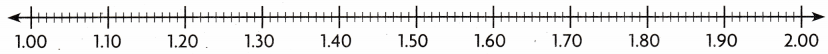Question 10.
1.18, 1.21, 1.05, 1.2
The given decimal numbers are: 1.18, 1.21, 1.05, and 1.2
Now,
The given number line is:Now,
The representation of the given decimal numbers on the number line is: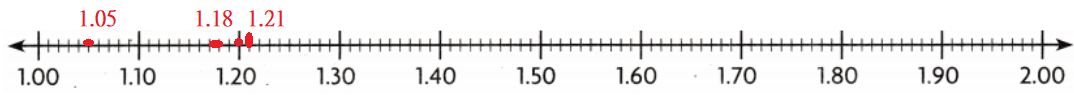So,
From the above number line,
We can observe that
The order of the decimal numbers from the least to the greatest is:
1.05, 1.18, 1.20, and 1.21
Hence, from the above,
We can conclude that
The order of the decimal numbers from the least to the greatest is:
1.05, 1.18, 1.20, and 1.21

Question 11.
1.43, 1.34, 1.4, 1.3
The given decimal numbers are: 1.43, 1.34, 1.4, and 1.3
Now,
The given number line is:Now,
The representation of the given decimal numbers on the number line is: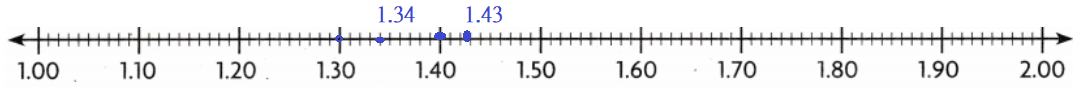So,
From the above number line,
We can observe that
The order of the decimal numbers from the least to the greatest is:
1.3, 1.34, 1.4, and 1.43
Hence, from the above,
We can conclude that
The order of the decimal numbers from the least to the greatest is:
1.3, 1.34, 1.4, and 1.43

Question 12.
Ken’s turtle competed in a 0.50-meter race. His turtle had traveled $$\frac{49}{100}$$ meter when the winning turtle crossed the finish line. What is $$\frac{49}{100}$$ written as a decimal?
(A) 0.09
(B) 0.49
(C) 0.40
(D) 4.90
It is given that
Ken’s turtle competed in a 0.50-meter race. His turtle had traveled $$\frac{49}{100}$$ meter when the winning turtle crossed the finish line
Now,
The representation of $$\frac{49}{100}$$ in the form of a decimal is: 0.49
Hence, from the above,
We can conclude that
The representation of $$\frac{49}{100}$$ in the form of a decimal is: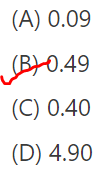Question 13.
Alex lives eight-tenths mile from Sarah. What are eight-tenths written as a decimal?
(A) 8.0
(B) 80.0
(C) 0.8
(D) 0.08
It is given that
Alex lives eight-tenths mile from Sarah
So,
The representation of eight-tenths in the form of a decimal is: 0.8
Hence, from the above,
We can conclude that
The representation of eight-tenths in the form of a decimal is:Question 14.
Which shows the decimals in order from greatest to least? You can use place value or a number line on your MathBoard.
(A) 4.67, 4.56, 4.55, 4.6
(B) 4.67, 4.6, 4.56, 4.55
(C) 4.55, 4.6, 4.67, 4.56
(D) 4.6, 4.55, 4.56,4.67
The order of the decimal numbers from the greatest to the least is:Question 15.
Elaine found the following in her pocket. Allow much money was in Elaine’s pocket?(A) $1.45 (B)$1.30
(C) $1.40 (D)$1.04
It is given that
Elaine found the following in her pocket
Now,
The given amount of money is:Now,
From the above,
We can observe that
The total amount of money Elaine has = (1 × $1) + (1 ×$0.25) + (2 × $0.1) =$1 + $0.25 +$0.2
= \$1.45
Hence, from the above,
We can conclude that
The total amount of money Elaine has is: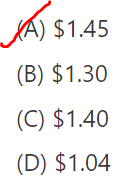Question 16.
Which fraction and decimal are shown by the point on the number line?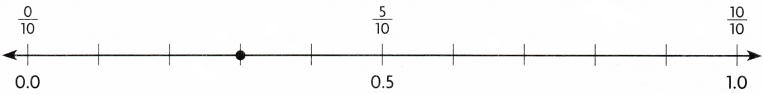(A) $$\frac{1}{10}$$; 0.1
(B) $$\frac{7}{10}$$; 0.7
(C) $$\frac{4}{10}$$; 0.4
(D) $$\frac{3}{10}$$; 0.3
The given number line is:Now,
The representation of the marked point on the given number line in the form of a fraction is: $$\frac{3}{10}$$
The representation of the marked point on the given number line in the form of a decimal is: 0.3
Hence, from the above,
We can conclude that
The fraction and decimal are shown by the point on the number line are: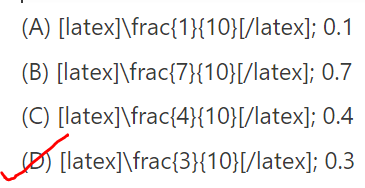Question 17.
Which fraction and decimal are shown by the point on the number line?(A) $$\frac{57}{100}$$; 0.57
(B) $$\frac{73}{100}$$; 0.73
(C) $$\frac{86}{100}$$; 0.86
(D) $$\frac{37}{100}$$; 0.37The representation of the marked point on the given number line in the form of a fraction is: $$\frac{73}{100}$$